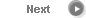#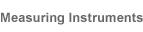# Precise Color Communication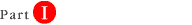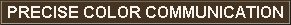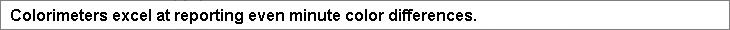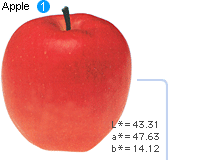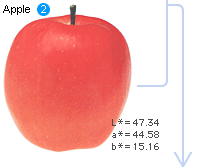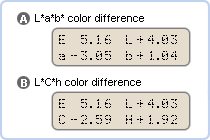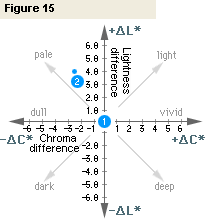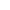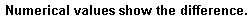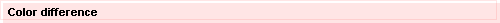Minute color differences are the biggest headache anywhere that color is used. But with a colorimeter, even minute color differences can be expressed numerically and easily understood. Let's use the L*a*b* and L*C*h color spaces to look at the color difference between two apples. Using apple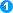's color (L*=43.31, a*=+47.63, b*=+14.12) as the standard, if we measure the difference of apple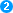's color (L*=47.34, a*=+44.58, b*=+15.16) from apple's color, we get the results shown in displays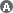and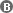below. The difference is also shown on the graph in Figure 13. The diagram of Figure 14 should make color difference in the L*a*b* color space easier to understand.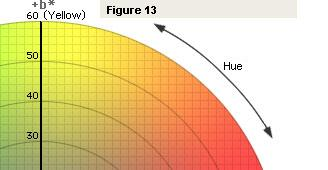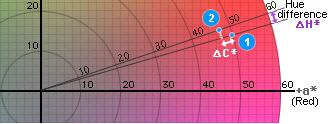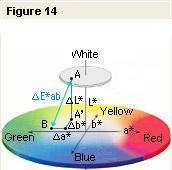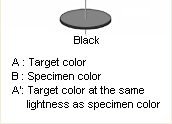In the L*a*b* color space, color difference can be expressed as a single numerical value, ∆E*ab, which indicates the size of the color difference but not in what way the colors are different. ∆E*ab is defined by the following equation:∆E*ab=[(∆L*)2 + (∆a*)2 + (∆b*)2]1/2
If we put the values ∆L*=+4.03, ∆a*=-3.05, and ∆b*=+1.04 from displayabove into this equation, we get ∆E*ab=5.16, which is the value shown in the upper left corner of display. If we measure the color difference between the two apples using the L*C*h color space, we get the results shown in displayabove. The value of ∆L* is the same as the value measured in the L*a*b* color space. ∆C*=-2.59, indicating that apple's color is less saturated. The hue difference between the two apples, ∆H* (defined by the equation
∆H*=[(∆E*ab)2 - (∆L*)2 - (∆C*)2]1/2), is +1.92, which if we look at Figure 13, means that the color of appleis closer to the +b* axis, and so is more yellow.
Although words are not as exact as numbers, we can use words to describe color differences. Figure 15 shows some of the terms used to describe differences in lightness and chroma; the terms shown in this figure indicate the direction of color difference, but unless an additional modifier (slightly, very, etc.) is used, they do not indicate the degree of color difference. If we look at the plotted values for the two apples, we see that we should say that the color of appleis "paler" than that of apple; since the chroma difference is not very great, we might also add a modifier, saying that appleis "slightly paler" to indicate the degree of difference.

• "∆" (delta) indicates difference.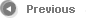11/13